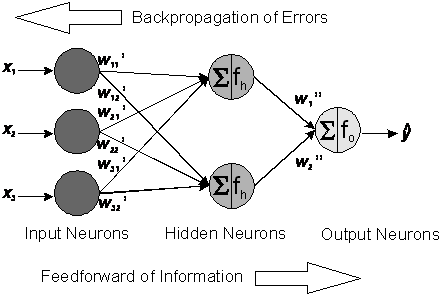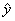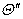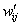Home News About Me Ph. D. Thesis Abstract Table of Contents 1. Introduction 2. Theory  Fundamentals of the Multivariate Data Analysis 2.1. Overview of the Multivariate Quantitative Data Analysis 2.2. Experimental Design 2.3. Data Preprocessing 2.4. Data Splitting and Validation 2.5. Calibration of Linear Relationships 2.6. Calibration of Nonlinear Relationships 2.7. Neural Networks  Universal Calibration Tools 2.7.1. Principles of Neural Networks 2.7.2. Topology of Neural Networks 2.7.3. Training of Neural Networks 2.8. Too Much Information Deteriorates Calibration 2.9. Measures of Error and Validation 3. Theory  Quantification of the Refrigerants R22 and R134a: Part I 4. Experiments, Setups and Data Sets 5. Results  Kinetic Measurements 6. Results  Multivariate Calibrations 7. Results  Genetic Algorithm Framework 8. Results  Growing Neural Network Framework 9. Results  All Data Sets 10. Results  Various Aspects of the Frameworks and Measurements 11. Summary and Outlook 12. References 13. Acknowledgements Publications Research Tutorials Downloads and Links Contact Search Site Map Print this Page### 2.7.1.   Principles of Neural Networks

Neural networks (NN) are parallel information processing systems consisting of a number of simple neurons (also called nodes or units), which are organized in layers and which are connected by links. The artificial neural networks imitate the highly interconnected structures of the brain and the nervous system of animals and humans whereby the neurons correspond to the cell bodies and the links are equivalent to the axons in biology. There are a number of different types of NN, whereby only multilayer feedforward neural networks are used and discussed in this study. An example of a multilayer feedforward neural network for three input variables x1, x2, x3 and one response variable y is shown in figure 1.figure 1:    Network elements of a multilayer feedforward backpropagation network.

The input variables are presented to the NN at the input units, which distribute the information by the connection links. Thereby the input variables are multiplied by the connection weights w'ij between the input and hidden layer. The hidden neurons sum the weighted signals from the input neurons and then project this sum on an activation function fh. The resulting activations of the hidden neurons are weighted by the connections w''j between the hidden and output neurons and sent to the output neuron(s). The output neuron also performs a summation and projection on its activation function fo. The output of this neuron is the estimated response. In the case of a single output neuron, the calculation of the estimated response can be summarized as:(11)

Thereby nv and nh are the number of input neurons and hidden neurons andandare the biases of the hidden and output neurons, which shift the transfer functions horizontally. The weightsandand the biasesandare the adjustable parameters, which are determined by a learning algorithm during the calibration (often called training or learning) and which are assigned random values before the calibration. During the training, calibration samples with known response variables y (concentrations) are passed through the network. Then, the error between the predicted responses and the known experimental responses is calculated and used to adjust the parameters of the net in a backpropagation step to minimize the error. Theses two steps form an epoch (also called learn cycle or learn step) and are repeated until an acceptable low error is reached. The learning algorithm tries to find an acceptable minimum on the error surface, whereby in most cases the absolute minimum of the error surface is not found.

 Page 44 © Frank Dieterle, 03.03.2019